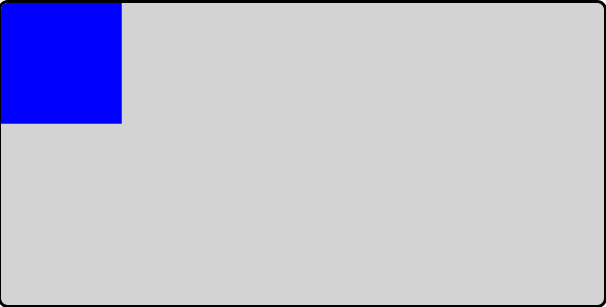Learn to Code via Tutorials on Repl.it!

← Back to all posts
Moving an HTML Canvas Element

# Moving an HTML Canvas Element

Moving an HTML canvas element can be extremely useful. You can make games and apps with moving and animated elements. Because the HTML canvas doesn't store which elements you create, you have to manually store them yourself. This tutorial will walk you through the steps of creating different functions and methods to move an element.

# Setting up the Canvas

First things first. Setting up the canvas element is simple. In the HTML editor, add a canvas element inside the body element:

``<canvas width="1000" height="500">An Error has Occured</canvas>``

This creates a new canvas 1000 pixels wide and 500 pixels tall. This completes everything on the html side.# Setting up the Java-Script Code

#### Defining Variables

Next, we need to set up some Java-Script code. In the JS editor, add a variable to get the canvas element and canvas context:

``````const canvas = document.getElementById("canvas");
const ctx = canvas.getContext("2d");``````

Some other variables to add are the canvas height and width. These can be used to wrap objects or set canvas boundaries.

``````const max_width = canvas.width;
const max_height = canvas.height;``````

We need a place to store our shapes (we will make them later). Create a new array to store them.

``var shapes = [];``

These are all of the initial variables we need.

#### Functions

Now we can start making some functions. We'll start with the shape constructor. This will create a shape object:

``````function Shape(x, y, w, h, f)
{
this.x = x || 0;
this.y = y || 0;
this.w = w || 1;
this.h = h || 1;
this.f = f || "black";
}``````

Next, we are going to create a method to draw a shape:

``````Shape.prototype.draw = function()
{
ctx.fillStyle = this.f;
ctx.fillRect(this.x, this.y, this.w, this.h);
}``````

In order to move a shape on the canvas, we need to clear the entire canvas, modify the shapes position and redraw everything. This function will clear the canvas and redraw the shapes in the given nested array:

``````function redraw(arrays)
{
ctx.clearRect(0, 0, max_width, max_height);
for (a in arrays)
{
for (b in a)
{
arrays[a][b].draw();
}
}
}``````

#### Drawing the Shape

Now we can make and move shapes. To make a shape, we can use the new Shape function:

``var box = new Shape(0, 0, 100, 100, "blue")``

We can change the x, y, width, height and color values later. Now we need to save the shape in the array we made.

``shapes.push(box)``Now comes the final part, moving the shape! We need to modify the x or y attributes of the shape and redraw the canvas. To modify the values, just add your_shape[value_name] = new_value. In this case:

``box["x"] += 1;``

Now that the attribute has been modified, redraw the canvas:

``redraw([shapes]);``

Repeatedly changing the shape attributes and then redrawing the canvas yields an animation. The animation above on the left uses the setInterval() to change the x attribute repeatedly and redraws the canvas. You can see the code for the animation here